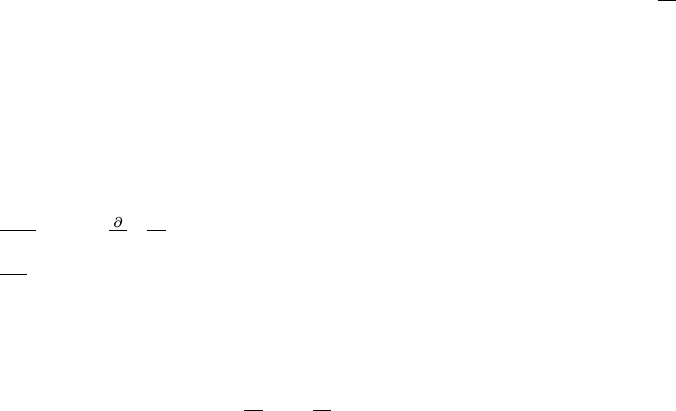# CSCI 2170 Lecture Notes - Lecture 13: Tangent Space, Partial Derivative, Level Set

16 views3 pages
School
Course
ProfessorMath 241 Chapter 13 Dr. Justin O. Wyss-Gallifent
§13.1 Functions of Several Variables
1. Deﬁnition: A function like f(x, y), f(x, y, z), g(s, t) etc.
2. Deﬁnition of the graph of a function of two variables and classic examples like: Plane, paraboloid,
cone, parabolic sheet, hemisphere.
3. Deﬁnition of level curve for f(x, y) and level surface for f(x, y, z).
4. Graphs of surfaces which are not necessarily functions: Sphere, ellipsoid, cylinder sideways
parabolic sheet like y=x2, double-cone.
§13.2 Limits and Continuity
1. Nothing much said other than lim
(x,y)(x0,y0)f(x, y) asks what f(x, y) approaches as (x, y) gets
closer to (x0, y0).
§13.3 Partial Derivatives
1. Defn: We can deﬁne the partial derivative of f(x, y)with respect to x, denoted f
x or fx, as the
derivative of ftreating all variables other than xas constant. Similarly for any variable for any
function.
2. For f(x, y) it turns out fxand fygive the slopes of the lines tangent to the graph of f(x, y) at
the point (x, y) in the positive xand positive ydirections respectively. A picture can clarify.
3. Higher derivatives will also be used but there are some points to note:
(a) fxy means (fx)yso ﬁrst take the derivative with respect to xand then y.
(b) 2f
x∂y means
x f
y so ﬁrst take the derivative with respect to yand then x.
(c) 2f
x2means xboth times.
(d) It turns out that 99% of the time the order doesn’t matter so for example fxy =fyx.
§13.4 The Chain Rule
1. Consider: For example if fis a function of xand ywhich are both functions of sand tthen really
fis a function of sand tand so f
s and f
t make sense. How to ﬁnd them?
2. The chain rule says:
(a) First draw the tree diagram.
(b) For each route from the starting to ending variable write down the product of the derivatives
along that path.
3. The chain rule is good for related rates problems when multiple rates are given and one rate is
needed.
Unlock document

This preview shows page 1 of the document.
Unlock all 3 pages and 3 million more documents.